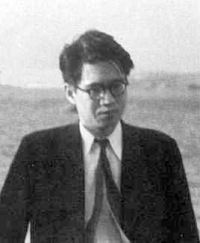# News from the world of maths: Mathematical moments

Wednesday, November 12, 2008

### Mathematical moments — Yutaka Taniyama

Born on the 12th of November 1927 in Kisai, Japan
Died on the 17th of November 1958 in Tokyo, JapanTaniyama's name is associated with one of the most famous problems in mathematics: Fermat's last theorem. The theorem says that for any whole number n strictly greater than 2, there are no three non-zero whole numbers x, y and z such that xn + yn = zn. Almost 400 years ago Fermat scribbled in the margin of a book that he had a proof for this assertion, which didn't fit in the margin. It wasn't until the 1990s that the theorem was finally proved by Andrew Wiles — and the proof definitely couldn't have been written in any margin!

Andrew Wiles didn't actually prove Fermat's last theorem, but a conjecture which now carries Taniyama's name. In 1955 Taniyama, who was working in algebraic number theory, posed a problem concerning so-called elliptic curves — these are curves defined by points in the plane whose co-ordinates satisfy a particular type of equation. Goro Shimura and André Weil mused over the question and formulated a conjecture: that every elliptic curve should come with a modular form, a mathematical object that is symmetrical in an infinite number of ways. This is now known as the Taniyama-Shimura-Weil conjecture.

The link to Fermat's last theorem was made around 30 years later, when the mathematician Ken Ribet realised that if Fermat's last theorem were false, then this would mean that a particular elliptic curve comes without a modular form. In other words, if Fermat's last theorem were false, then the Taniyama-Shimura-Weil conjecture would be false also. Reformulating this yet again, if the Taniyama-Shimura-Weil conjecture is true, then so is Fermat's last theorem. What Andrew Wiles proved is that the Taniyama-Shimura-Weil conjecture is indeed true for a class of examples that is sufficient to prove Fermat's last theorem. QED.

In 1958, just days after his 31st birthday and not long before he was meant to get married, Taniyama committed suicide. His explanation was this: "Until yesterday I had no definite intention of killing myself. ... I don't quite understand it myself, but it is not the result of a particular incident, nor of a specific matter."

To find out more about Fermat's last theorem and its proof, read the Plus articles Fermat's last theorem and Andrew Wiles and How maths can make you rich and famous.

At 10:34 AM,Kuldeep said...